Next: Count rate predictions for Up: Flux conversions Previous: General considerations

## Count rate predictions for simple spectra

This section provides a set of graphs which can be used to estimate the WFC count rate in each of the scientific filters as a function of spectral parameters and for a range of different spectral models. The models included are:

1. power law (Figure 12.3)
2. optically thin plasma (Figure 12.4; cosmic abundance spectra computed following Raymond and Smith, 1977, ApJS, 35, 419)
3. black-body (Figure 12.5)
4. two different model atmospheres (mainly applicable to white dwarfs; Figures 12.6and 12.7) - these are discussed in § 12.3.3below.

For all spectral models, and for each of the scientific filters as listed in Table 5.3, the graphs show the expected WFC count rate as a function of temperature (or energy index in the case of power law) for a set of column densities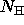varying from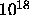tocm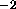. The absorption incorporated uses the cross-sections given by Morrison and McCammon (1983, ApJ, 270, 119) for energies above 0.03 keV (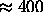Å), extrapolated to lower energies using the cross-sections given by Cruddace et al. (1974, ApJ, 187, 497).

The plots are normalized to give the count rates for a nominal unabsorbed flux of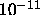ergs cms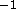in the energy range 0.02 - 2 keV. (Note that this is substantially larger than the actual pass-band of the WFC which is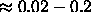keV, depending on filter choice. We use this band to facilitate comparison with XRT predictions, or with EXOSAT CMA results.) Thus the estimated count rate for the models is calculated by multiplying the plotted conversion factor (CF) by the estimated unabsorbed source flux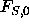(in the 0.02 - 2 keV band) in units ofergs cms, i.e.,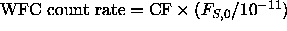.

The unabsorbed flux in this context means the flux in the passband assuming no interstellar absorption, i.e., if the source has an assumed spectrum S(E) and interstellar absorption is modelled as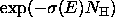where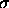is the photoelectric cross-section andthe column density, the absorbed and unabsorbed source fluxes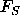andare defined as follows: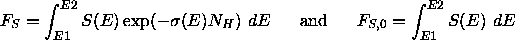respectively, where E1 and E2 are the energy limits of the passband.Next: Count rate predictions for Up: Flux conversions Previous: General considerations

Michael Arida
Tue Jun 11 16:18:41 EDT 1996